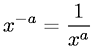Equations > Algebra > Exponents/Powers > Negative Exponent Definition

### Negative Exponent DefinitionLatex Code:

MathML Code:

 ${x}^{-a}=\frac1{x}^{a}$

MathType 5.0: